# Privacy and tomography

I ran across the image below  when I was searching for something else, and it made me think of a few issues in data privacy.The green lights cut across the man’s body like tomographic imaging. No one of these green lines would be identifiable, but maybe the combination of the lines is.

We can tell it’s a young man in the image, someone in good shape. But is this image identifiable?

It’s identifiable to the photographer. It’s identifiable to the person in the photo.

If hundreds of men were photographed under similar circumstances, and we knew who those men were, we could probably determine which of them is in this particular photo.

The identifiability of the photo depends on context. The HIPAA Privacy Rule acknowledges that the risk of identification of individuals in a data set depends on who is receiving the data and what information the recipient could reasonably combine the data with. The NSA could probably tell who the person in the photo is; I cannot.

Making photographs like this available is not a good idea if you want to protect privacy, even if its debatable whether the person in the photo can be identified. Other people photographed under the same circumstances might be easier to identify.

Differential privacy takes a more conservative approach. Differential privacy advocates argue that deidentification procedures should not depend on what the recipient knows. We can never be certain what another person does or doesn’t know, or what they might come to know in the future.

Conventional approaches to privacy consider some data more identifiable than other data. Your phone number and your lab test results from a particular time and place are both unique, but the former is readily available public information and the latter is not. Differential privacy purists would say both kinds of data should be treated identically. A Bayesian approach might be to say we need to multiply each risk by a prior probability: the probability of an intruder looking up your phone number is substantially greater than the probability of the intruder accessing your medical records.

Some differential privacy practitioners make compromises, agreeing that not all data is equally sensitive. Purists decry this as irresponsible. But if the only alternatives are pure differential privacy and traditional approaches, many clients will choose the latter, even though an impure approach to differential privacy would have provided better privacy protection.

 Photo by Caspian Dahlström on Unsplash

# Privacy implications of hashing data

Cryptographic hash functions are also known as one-way functions because given an input x, one can easily compute its hashed value f(x), but it is impractical to recover x from knowing f(x).

However, if we know that x comes from a small universe of possible values, our one-way function can effectively become a two-way function, i.e. it may be possible to start with f(x) and recover x using a rainbow table attack.

It’s possible to defend against a rainbow attack yet still leak information about the probability distribution of values of x given f(x).

For privacy protection, hashing is better than not hashing. Keyed hashing is better than unkeyed hashing. But even keyed hashing can leak information.

## Rainbow tables

Suppose a data set contains SHA-256 hashed values of US Social Security Numbers (SSNs). Since SSNs have 10 digits, there are 10 billion possible SSNs. It would be possible to hash all possible SSNs and create a lookup table, known as a rainbow table. There are three things that make the rainbow table attack possible in this example:

1. The range of possible inputs is known and relatively small.
2. The hashing algorithm is known.
3. The hashing algorithm can be computed quickly.

There are a couple ways to thwart a rainbow table attack. Assuming we have no control of (1) above, we can alter (2) or (3).

### Keyed hashing

A way to alter (2) is to use a keyed hash algorithm. For example, if we XOR the SSNs with a key before applying SHA-256, an attacker cannot construct a rainbow table without knowing the key. The attacker may know the core hash algorithm we are using, but they do not know the entire algorithm because the key is part of the algorithm.

### Expensive hashing

A way to alter (3) is to use hashing algorithms that are expensive to compute, such as Argon2. The idea is that such hash functions can be computed quickly enough for legitimate use cases, but not for brute-force attacks.

The time required to create a rainbow table is the product of the time to compute a single hash and the size of the set of possible inputs. If it took an hour to compute a hash value, but there are only 10 possible values, then an attacker could compute a rainbow table in 10 hours.

## Leaking attribute probabilities

Now suppose we’re using a keyed hashing algorithm. Maybe we’re paranoid and use an expensive hashing algorithm on top of that. What can go wrong now?

If we’re hashing values that each appear one time then we’re OK. If we apply a keyed hash to primary keys in a database, such as patient ID, then the hashed ID does not reveal the patient ID. But if we hash attributes associated with that patient ID, things are different.

### Frequency analysis

Suppose US state is an attribute in your database, and you hash this value. No matter how secure and how expensive your hash algorithm is, each state will have a unique hash value. If the database contains a geographically representative sample of the US population, then the hashed state value that appears most often is probably the most populous state, i.e. California. The second most common hashed state value probably corresponds to Texas.

Things are fuzzier on the low end. The hashed state value appearing least often may not correspond to Wyoming, but it very likely does not correspond to Florida, for example.

In short, you can infer the state values using the same kind of frequency analysis you’d use to solve a simple substitution cipher in a cryptogram puzzle. The larger the data set, the more closely the empirical order will likely align with the population order.

### Maybe this is OK?

Frequency analysis makes it possible to infer (with some uncertainty) the most common values. But the most common values are also the least informative. Knowing someone is from California narrows down their identity less than knowing that they are from Wyoming.

Frequency analysis is less specific for less common values. It might not tell you with much confidence that someone is from Wyoming, but it might tell you with some confidence that they come from a low-population state. However, since there are several low-population states, knowing someone is from such a state, without knowing which particular state, isn’t so informative.

Data privacy depends on context. Knowing what state someone is likely from may or may not be a problem depending on what other information is available.

# Burr distribution

Irving Burr came up with a set of twelve probability distributions known as Burr I, Burr II, …, Burr XII. The last of these is by far the best known, and so the Burr XII distribution is often referred to simply as the Burr distribution . See the next post for the rest of the Burr distributions.

Cumulative density functions (CDFs) of probability distributions don’t always have a closed form, but it’s convenient when they do. And the CDF of the Burr XII distribution has a particularly nice closed form:You can also add a scale parameter the usual way.

The flexibility of the Burr distribution isn’t apparent from looking at the CDF. It’s obviously a two-parameter family of distributions, but not all two-parameter families are equally flexible. Flexibility is a fuzzy concept, and there are different notions of flexibility for different applications. But one way of measuring flexibility is the range of (skewness, kurtosis) values the distribution family can have.

The diagram below  shows that the Burr distribution can take on a wide variety of skewness and kurtosis combinations, and that for a given value of skewness, the Burr distribution has the largest kurtosis of common probability distribution families. (Note that the skewness axis increases from top to bottom.)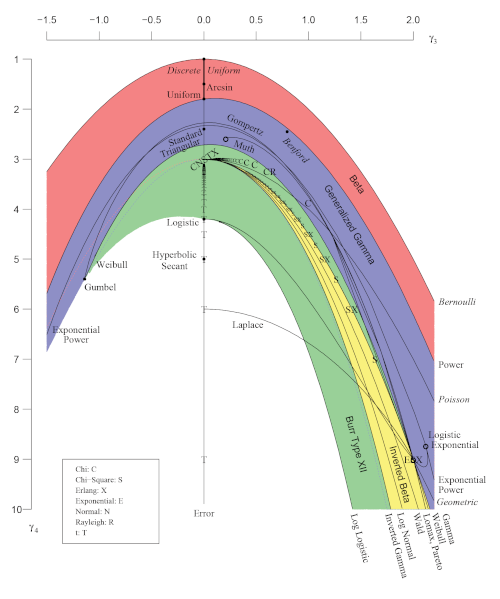The green area at the bottom of the rainbow belongs to the Burr distribution. The yellow area is a subset of the green area.

 In economics the Burr Type XII distribution is also known as the Singh-Maddala distribution.

 Vargo, Pasupathy, and Leemis. Moment-Ratio Diagrams for Univariate Distributions. Journal of Quality Technology. Vol. 42, No. 3, July 2010. The article contains a grayscale version of the image, and the color version is available via supplementary material online.

# Maxwell-Boltzmann and Gamma

When I shared an image from the previous post on Twitter, someone who goes by the handle Nonetheless made the astute observation that image looked like the Maxwell-Boltzmann distribution. That made me wonder what 1/Γ(x) would be like turned into a probability distribution, and whether it would be approximately like the Maxwell-Boltzmann distribution.

(Here I’m looking at something related to what Nonetheless said, but different. He was looking at my plot of the error in approximating 1/Γ(x) by partial products, but I’m looking at 1/Γ(x) itself.)

## Making probability distributions

You can make any non-negative function with a finite integral into a probability density function by dividing it by its integral. So we can define a probability density functionwhereand so c = 0.356154. (The integral cannot be computed in closed form and so has to be evaluated numerically.)

Here’s a plot of f(x).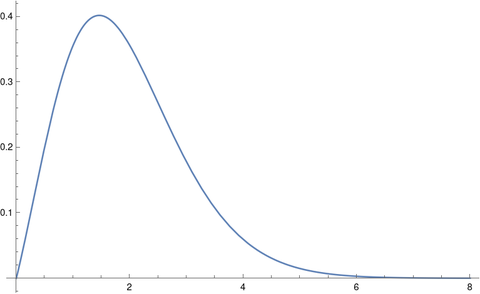Note that we’re doing something kind of odd here. It’s common to see the gamma function in the definition of probability distributions, but the function is always evaluated at distribution parameters, not at the free variable x. We’ll give an example of this shortly.

## Maxwell-Boltzmann distribution

The Maxwell-Boltzmann distribution, sometimes called just the Maxwell distribution, is used in statistical mechanics to give a density function for particle velocities. In statistical terminology, the Maxwell-Boltzmann distribution is a chi distribution  with three degrees of freedom and a scale parameter σ that depends on physical parameters.

The density function for a Maxwell-Boltzmann distribution with k degrees of freedom and scale parameter σ has the following equation.Think of this as xk-1 exp(-x² / 2σ²) multiplied by whatever you have to multiply it by to make it integrate to 1; most of the complexity in the definition is in the proportionality constant. And as mentioned above, the gamma function appears in the proportionality constant.

For the Maxwell-Boltzmann distribution, k = 3.

## Lining up distributions

Now suppose we want to compare the distribution we’ve created out of 1/Γ(x) with the Maxwell-Boltzman distribution. One way of to do this would be to align the two distributions to have their peaks at the same place. The mode of a chi random variable with k degrees of freedom and scale σ isand so for a given k we can solve for σ to put the mode where we’d like.

For positive values, the minimum of the gamma function, and hence the maximum of its reciprocal, occurs at 1.46163. As with the integral above, this has to be computed numerically.

For the Maxwell-Boltzmann distribution, i.e. when k = 3, we don’t get a very good fit.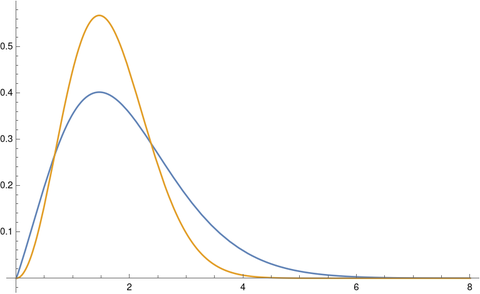The tails on the chi distribution are too thin. If we try again with k = 2 we get a much better fit.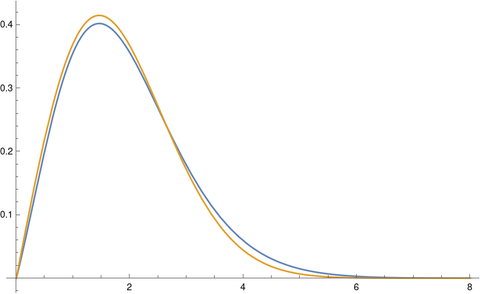You get an even better fit with k = 1.86. The optimal value of k would depend on your criteria for optimality, but k = 1.86 looks like a good value just eyeballing it.

 This is a chi distribution, not the far more common chi-square distribution. The first time you see this is looks like a typo. If X is a random variable with a chi random variable with k degrees of freedom then X² has a chi-square distribution with k degrees of freedom.

# Surprisingly not that surprisingWorld record marathon times have been falling in increments of roughly 30 seconds, each new record shaving roughly 30 seconds off the previous record. If someone were to set a new record, taking 20 seconds off the previous record, this would be exciting, but not suspicious. If someone were to take 5 minutes off the previous record, that would be suspicious.

One way to quantify how surprising a new record is would be to divide its margin of improvement over the previous margin of improvement. That is, given a new record y, and previous records y1 and y2, we can calculate an index of surprise by

r = (yy1) / (y1y2)

In  the authors analyze this statistic and extensions that take into account more than just the latest two records under technical assumptions I won’t get into here.

A p-value for the statistic R is given by

Prob(R > r) = 2/(r + 2).

You could think of this as a scale of surprise, with 0 being impossibly surprising and 1 being completely unremarkable.

There are multiple reasons to take this statistic with a grain of salt. It is an idealization based on assumptions that may not even approximately hold in a particular setting. And yet it is at least a useful rule of thumb.

The current marathon record beat the previous record by by 30 seconds. The previous margin of improvement was 78 seconds. This gives a value of r equal to 0.385 and a corresponding p-value of 0.84. This says the current record is impressive but statistically unremarkable. An improvement of 5 minutes, i.e. 300 seconds, would result in a p-value of 0.17, which is notable but not hard evidence cheating. 

The assumptions in  do not apply to marathon times, and may not apply to many situations where the statistic above nevertheless is a useful rule of thumb. The ideas in the paper could form the basis of a more appropriate analysis customized for a particular application.

Reports of a new record in any context are usually over-hyped. The rule of thumb above gives a way to gauge for yourself whether you should share the report’s excitement. You shouldn’t read too much into it, like any rule of thumb, but it at least gives a basis for deciding whether something deserves closer attention.

## More rule of thumb posts

 Andrew R. Solow and Woollcott Smith. How Surprising Is a New Record? The American Statistician, May, 2005, Vol. 59, No. 2, pp. 153-155

 I haven’t done the calculations, but I suspect that if you used the version of the index of surprise in  that takes into account more previous records, say the last 10, then you’d get a much smaller p-value.

The image at the top of the post is of Eliud Kipchoge, current marathon world record holder. Image under Creative Commons license. source.

# Big correlations and big interactions

An outcome cannot be highly correlated with a large number of independent predictors.

This observation has been called the piranha problem. Predictors are compared to piranha fish. If you have a lot of big piranhas in a small pond, they start eating each other. If you have a lot of strong predictors, they predict each other.

In  the authors quantify the piranha effect several ways. I’ll just quote the first one here. See the paper for several other theorems and commentary on their implications.

If X1, …, Xp, y are real-valued random variables with finite non-zero variance, thenSo if the left side is large, either because p is large or because some of the correlations are large, then the right side is also large, and so the sum of the interaction terms is large.

## Related posts

. The piranha problem: large effects swimming in a small pond. Available on arxiv.

# Design of experiments and design theoryDesign of experiments is a branch of statistics, and design theory is a branch of combinatorics, and yet they overlap quite a bit.

It’s hard to say precisely what design theory is, but it’s consider with whether objects can be arranged in certain ways, and if so how many ways this can be done. Design theory is pure mathematics, but it is of interest to people working in ares of applied mathematics such as coding theory and statistics.

Here’s a recap of posts I’ve written recently related to design of experiments and design theory.

## Design of Experiments

A few weeks ago I wrote about fractional factorial design. Then later I wrote about response surface models. Then a diagram from central composite design, a popular design in response surface methodology, was one the diagrams in a post I wrote about visually similar diagrams from separate areas of application.

I wrote two posts about pitfalls with A/B testing. One shows how play-the-winner sequential testing has the same problems as Condorcet’s voter paradox, with the order of the tests potentially determining the final winner. More seriously, A/B testing cannot detect interaction effects which may be critical.

## ANSI and Military Standards

There are several civilian and military standards related to design of experiments. The first of these was MIL-STD-105. The US military has retired this standard in favor of the civilian standard ASQ/ANSI Z1.4 which is virtually identical.

Similarly, the US military standard MIL-STD-414 was replaced by the very similar civilian standard ASQ/ANSI Z1.9. This post looks at the mean-range method for estimating variation which these two standards reference.

## Design Theory

I wrote a couple posts on Room squares, one on Room squares in general and one on Thomas Room’s original design now known as a Room square. Room squares are used in tournament designs.

I wrote a couple posts about Costas arrays, an introduction and a post on creating Costas arrays in Mathematica.

## Latin Squares

Latin squares and Greco-Latin squares a part of design theory and a part of design of experiments. Here are several posts on Latin and Greco-Latin squares.

# Three diagrams

This post will give examples of three similar diagrams that occur in three dissimilar areas: design of experiments, finite difference methods for PDEs, and numerical integration.

## Central Composite Design (CCD)

The most popular design for fitting a second-order response surface is the central composite design or CCD. When there are two factors being tested, the combinations of the two factors are chosen according to the following diagram, with the value at the center being used for several replications, say four.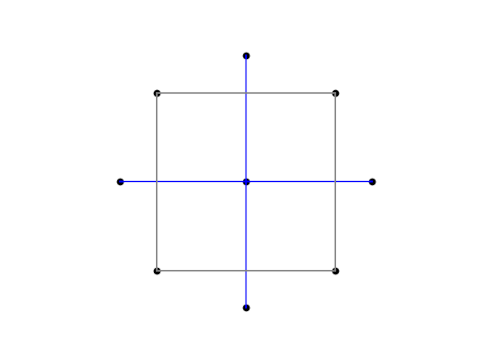The variables are coded so that the corners of the square have both coordinates equal to ±1. The points outside the square, the so-called axial points, have one coordinate equal to 0 and another equal to ±√2.

## Finite difference method

The star-like arrangement of points with the middle being weighted more heavily is reminiscent of the five-point finite difference template for the Laplacian. Notice that the center point is weighted by a factor of -4.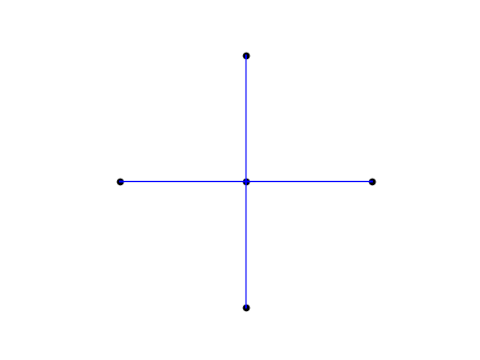In the finite difference method, this pattern of grid points carries over to a pattern of matrix coefficients.

## Integrating over a disk

The CCD grid looks even more like a numerical integration pattern for a function f defined over a disk of radius h. See A&S equation 25.4.61.The center of the circle has weight w = 1/6. The four points on the circle are located at (±h, 0) and (0, ±h) and have weight w = 1/24. The remaining points are located at (±h/2, ±h/2) and have weight w = 1/6.

# Another problem with A/B testing: interaction effectsThe previous post looked at a paradox with A/B testing: your final result may depend heavily on the order of your tests. This post looks at another problem with A/B testing: the inability to find interaction effects.

Suppose you’re debating between putting a photo of a car or a truck on your website, and you’re debating between whether the vehicle should be red or blue. You decide to use A/B testing, so you test whether customers prefer a red truck or a blue truck. They prefer the blue truck. Then you test whether customers prefer a blue truck or a blue car. They prefer the blue truck.

Maybe customers would prefer a red car best of all, but you didn’t test that option. By testing vehicle type and color separately, you didn’t learn about the interaction of vehicle type and color. As Andrew Gelman and Jennifer Hill put it ,

Interactions can be important. In practice, inputs that have large main effects also tend to have large interactions with other inputs. (However, small main effects do not preclude the possibility of large interactions.)

Notice that sample size is not the issue. Suppose you tested the red truck against the blue truck with 1000 users and found that 88.2% preferred the blue truck. You can be quite confident that users prefer the blue truck to the red truck. Suppose you also used 1000 users to test the blue truck against the blue car and this time 73.5% preferred the blue truck. Again you can be confident in your results. But you failed to learn something that you might have learned if you’d split 100 users between four options: red truck, blue truck, red car, blue car.

## Experiment size

This is an example of a factorial design, testing all combinations of the factors involved. Factorial designs seem impractical because the number of combinations can grow very quickly as the number of factors increases. But if it’s not practical to test all combinations of 10 factors, for example, that doesn’t mean that it’s impractical to test all combinations of two factors, as in the example above. It is often practical to use a full factorial design for a moderate number of factors, and to use a fractional factorial design with more factors.

If you only test one factor at a time, you’re betting that interaction effects don’t matter. Maybe you’re right, and you can optimize your design by optimizing each variable separately. But if you’re wrong, you won’t know.

## Agility

The advantage of A/B tests is that they can often be done rapidly. Blue or red? Blue. Car or truck? Truck. Done. Now let’s test something else.

If the only options were between a rapid succession of tests of one factor at a time or one big, complicated statistical test of everything, speed might win. But there’s another possibility: a rapid succession of slightly more sophisticated tests.

Suppose you have 9 factors that you’re interested in, and you understandably don’t want to test several replications of 29 = 512 possibilities. You might start out with a (fractional) factorial design of 5 of the factors. Say that only one of these factors seems to make much difference, no matter what you pair it with. Next you do another experiment testing 5 factors at a time, the winner of the first experiment and the 4 factors you haven’t tested yet. This lets you do two small experiments rather than one big one.

Note that in this example you’re assuming that the factors that didn’t matter in the first experiment wouldn’t have important interactions with the factors in the second experiment. And your assumption might be wrong. But you’re making an educated guess, based on data from the first experiment. This is less than ideal, but it’s better than the alternative of testing every factor one at a time, assuming that no interactions matter. Assuming that some interactions don’t matter, based on data, is better than making a blanket assumption that no interactions matter, based on no data.

Testing more than one factor at a time can be efficient for screening as well as for finding interactions. It can help you narrow in on the variables you need to test more thoroughly.

## Related posts

 Andrew Gelman and Jennifer Hill. Data Analysis Using Regression and Multilevel/Hierarchical Models. Cambridge University Press, 2007.

# ASQ/ANSI Z1.4 sampling procedures

I mentioned the other day that the US military standard MIL-STD-105 for statistical sampling procedures lives on in the ASQ/ANSI standard Z1.4. The Department of Defense cancelled their own standard in 1995 in favor of adopting civilian standards, in particular ASQ/ANSI Z1.4.

There are two main differences between military standard and its replacement. First, the military standard is free and the ASNI standard costs \$199. Second, the ASNI standard has better typography. Otherwise the two standards are remarkably similar.

For example, the screen shot from MIL-STD-105 that I posted the other day appears verbatim in ASQ/ASNI Z1.4, except with better typesetting. The table even has the same name: “Table II-B Single sampling plans for tightened inspection (Master table).” Since the former is public domain and the latter is copyrighted, I’ll repeat my screenshot of the former.Everything I said about the substance of the military standard applies to the ASNI standard. The two give objective, checklist-friendly statistical sampling plans and acceptance criteria. The biggest strength and biggest weakness of these plans is the lack of nuance. One could create more sophisticated statistical designs that are more powerful, but then you lose the simplicity of a more regimented approach.

A company could choose to go down both paths, using more informative statistical models for internal use and reporting results from the tests dictated by standards. For example, a company could create a statistical model that takes more information into account and use it to assess the predictive probability of passing the tests required by sampling standards.